Scheduling parameters are used during the running of DataWorks nodes. The scheduling parameters are automatically replaced with specific values based on the data timestamps of the nodes and the value formats of the scheduling parameters. This implements dynamic parameter settings during the running of nodes. You can configure scheduling parameters for a node in the Parameters field of the General section on the Properties tab. This topic describes the types of scheduling parameters and the precautions for using scheduling parameters. It also provides some typical configuration examples of scheduling parameters.

## Precautions

• You can configure scheduling parameters for an auto triggered node and deploy it to the production environment. This way, the scheduling system can automatically replace the scheduling parameters based on the data timestamp of the node. You can click Run or Run with Parameters on the DataStudio page to perform a test for the node. However, the scheduling system does not automatically replace the parameters during the test. You must manually assign values to the scheduling parameters that you want to reference in the code of the node.

For example, you configure the scheduling parameter bdp.system.bizdate for an auto triggered node. During the running of the node, the scheduling system automatically replaces this parameter with the specific data timestamp. However, if you click Run on the DataStudio page to perform a test for the node, the parameter is not automatically replaced. In this case, you must manually assign a constant to the parameter in the code of the node. For more information, see Test scheduling parameters in the development environment.

• If you want to check whether a scheduling parameter is replaced as expected, you must perform smoke testing in the development environment. For more information, see Test scheduling parameters in the development environment.
• If you changed the variables in the code or you want to re-assign constants to the variables, you must click Run with Parameters.

Each time you click Run with Parameters to run a node, the system prompts you to assign constants to the variables in the code. This ensures that the variable values in the code are correct each time the system runs the node. If you click Run to run a node for the first time, the system prompts you to assign constants to the variables. In subsequent running, you are no longer required to assign constants to the variables, and the constants assigned the first time are used. As a result, the constants may be invalid for the subsequent running.

• Before you use \${...} and \$[...] parameters, take note of the following items:
• If you want to specify a time that is N hours or minutes ago, use a \$[...] parameter.

\${...} parameters are accurate only to the day. Therefore, you cannot use a \${...} parameter to specify a time that is accurate to the hour, minute, or second, such as \${yyyy-mm-dd-1/24}. If you want to specify a time that is accurate to the second, we recommend that you use a \$[...] parameter, such as \$[yyyy-mm-dd-1-1/24].

• If you want to specify a time that is N years or months ago, use a \${...} parameter.

You cannot use a \$[...] parameter to specify a calculation-based time, such as \$[yyyy-N] or \$[mm-N]. If you want to specify a calculation-based time, we recommend that you use a \${...} parameter, such as \${yyyy-N} or \${mm-N}.

## Types of scheduling parameters

Scheduling parameters are classified into built-in system variables and custom parameters based on whether value assignment is required. We recommend that you use custom parameters.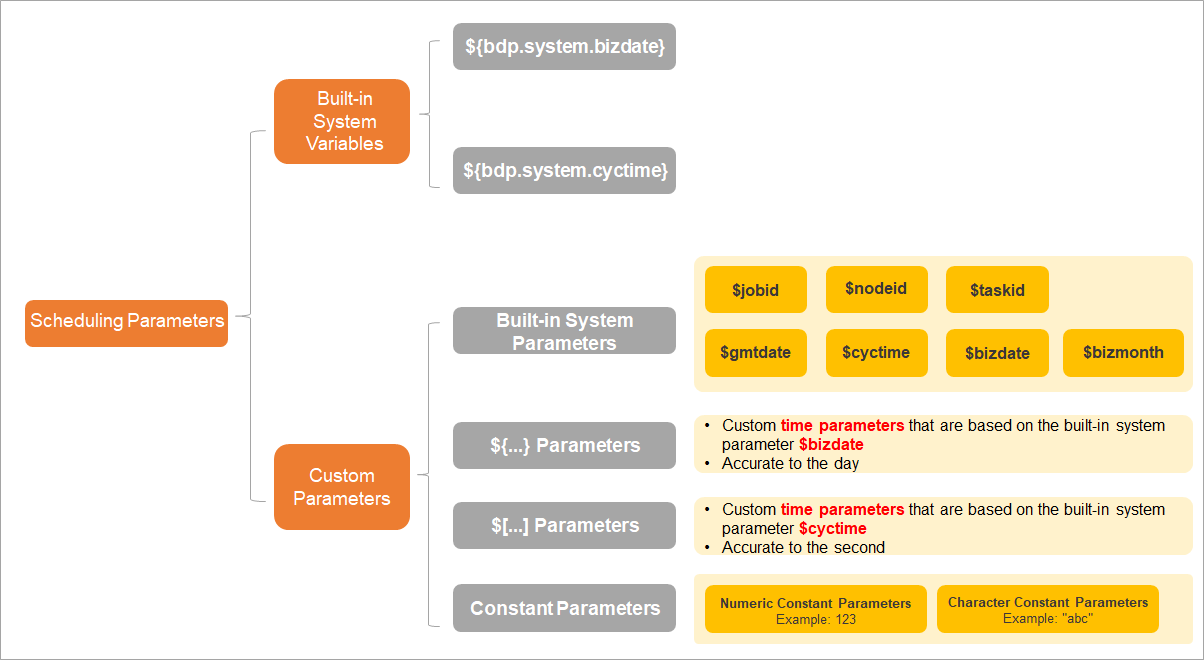## Built-in system variables

• Built-in system variables
Built-in system variable Description Remarks
\${bdp.system.bizdate} The data timestamp of an instance. By default, the date indicated by the data timestamp is one day before the time when the instance is scheduled to run. Default format: yyyymmdd. The value can be accurate to the day.

The following formula shows the relationship between the two built-in system variables: \${bdp.system.cyctime} = \${bdp.system.bizdate} + 1. This indicates that the date indicated by the data timestamp of a node is one day before the time when the node is scheduled to run.

\${bdp.system.cyctime} The time when the instance is scheduled to run. Default format: yyyymmddhh24miss. The value can be accurate to the second.
• Assignment and reference requirements
You can reference built-in system variables in the code of your node without the need to assign values to them.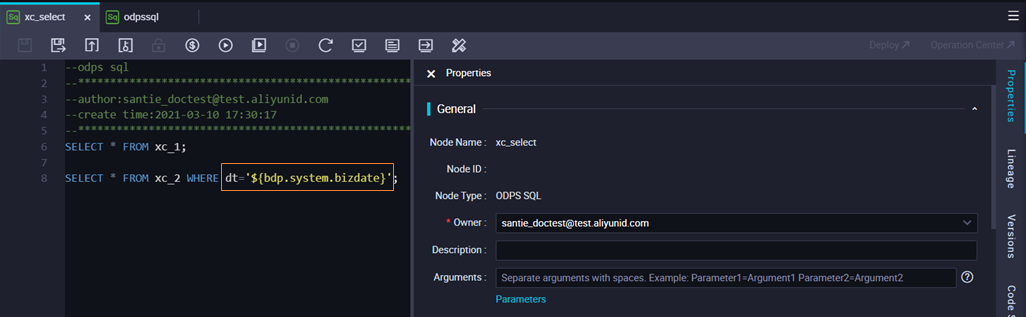## Custom parameters: built-in system parameters

• Parameters
Table 1. Built-in system parameters
Built-in system parameter Description
\$bizdate The data timestamp of a node, in the format of yyyymmdd.

This parameter is widely used. By default, the date indicated by the data timestamp of a node is one day before the time when the node is scheduled to run.

\$cyctime The time when the node is scheduled to run, in the format of yyyymmddhh24miss.
\$gmtdate The current date, in the format of yyyymmdd.

By default, the value of this parameter is the current date. During data backfill, the input value is one day after the date indicated by the data timestamp.

\$bizmonth The month indicated by the data timestamp, in the format of yyyymm.
• If the month indicated by a data timestamp is the current month, the value of this parameter is one month before the month indicated by the data timestamp.
• If the month indicated by a data timestamp is not the current month, the value of this parameter is the month indicated by the data timestamp.
\$jobid The ID of the workflow to which the node belongs.
\$nodeid The ID of the node.
\$taskid The ID of the node instance.
• Assignment and reference requirements for ODPS SQL nodes, batch synchronization nodes, and PyODPS nodes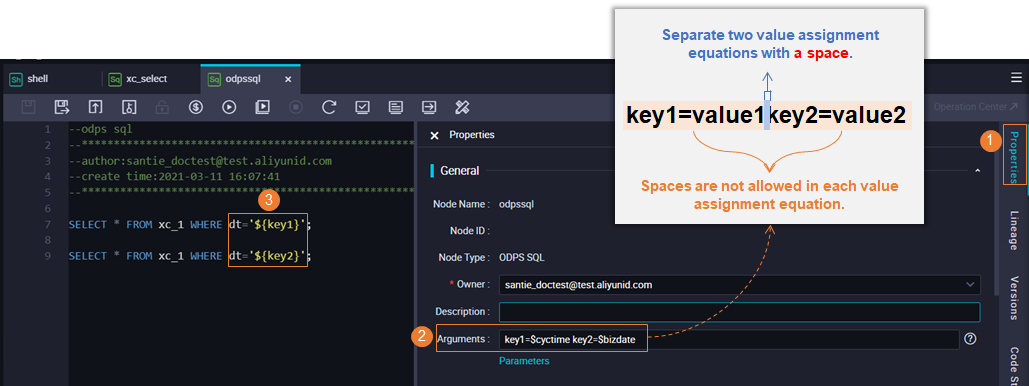• You must assign built-in system parameters to variables in the Parameters field of the General section on the Properties tab. Separate multiple assignment equations with spaces.
• Assign built-in system parameters in the `key1=value1 key2=value2` format. `key1` and `key2` are custom variables. `value1` and `value2` are built-in system parameters. For more information about the built-in system parameters provided by DataWorks, see Table 1.
• Reference built-in system parameters in the following ways:
• ODPS SQL nodes, E-MapReduce (EMR) nodes, and batch synchronization nodes

Directly reference `\${key1}` or `\${key2}` in code. key1 and key2 are custom variables. Example: `dt=\${key1}`.

• PyODPS nodes

Add a dictionary object named args to the global variable: `args=['key1'] args=['key2']`. key1 and key2 are custom variables.

• Assignment and reference requirements for common Shell nodes
• Assign built-in system parameters in the `value1 value2` format. `value1` and `value2` are built-in system parameters. For more information about the built-in system parameters provided by DataWorks, see Table 1.
• Reference built-in system parameters in the following way:

Reference variables \$1, \$2, ... in code.

Note For more information about the assignment and reference requirements for EMR Shell nodes and EMR Spark Shell nodes, see the assignment and reference requirements for ODPS SQL nodes.

## Custom parameters: \${...} parameters

• Parameter definition
\${...} parameters are custom time parameters that are based on the built-in system parameter \$bizdate. You can use the following items to customize such a time parameter:
• yyyy: indicates the four-digit year, which is the year in the value of the \$bizdate parameter.
• yy: indicates the two-digit year, which is the year in the value of the \$bizdate parameter.
• mm: indicates the month, which is the month in the value of the \$bizdate parameter.
• dd: indicates the day, which is the day in the value of the \$bizdate parameter.
You can specify one or more of the preceding items based on your requirements, such as \${yyyy}, \${yyyymm}, \${yyyymmdd}, and \${yyyy-mm-dd}.
Note The \$bizdate parameter is accurate to the day. Therefore, \${...} parameters are also accurate only to the day. This way, you cannot use a \${...} parameter to specify a time that is accurate to the hour, minute, or second, such as \${yyyy-mm-dd-1/24}. If you want to specify a time that is accurate to the second, we recommend that you use a \$[...] parameter, such as \$[yyyy-mm-dd-1-1/24].
• Assignment and reference requirements for ODPS SQL nodes, batch synchronization nodes, PyODPS nodes, and EMR nodes• You must assign custom parameters to variables in the Parameters field of the General section on the Properties tab. After you assign custom parameters to variables, you can reference the variables in the code of your node.
• Assign custom parameters in the `key1=value1 key2=value2` format. `key1` and `key2` are custom variables. `value1` and `value2` are custom parameters. The following table lists the examples of the custom parameters.
Interval that you want to add or remove Custom parameter
N years later \${yyyy+N}
N years ago \${yyyy-N}
N months later \${yyyymm+N}
N months ago \${yyyymm-N}
N weeks later \${yyyymmdd+7*N}
N weeks ago \${yyyymmdd-7*N}
N days later \${yyyymmdd+N}
N days ago \${yyyymmdd-N}
N days after the specified date \${yyyymmdd+N}
N days before the specified date \${yyyymmdd-N}
• Reference built-in system parameters in the following ways:
• ODPS SQL nodes, E-MapReduce (EMR) nodes, and batch synchronization nodes

Directly reference `\${key1}` or `\${key2}` in code. key1 and key2 are custom variables. Example: `dt=\${key1}`.

• PyODPS nodes

Add a dictionary object named args to the global variable: `args=['key1'] args=['key2']`. key1 and key2 are custom variables.

• Assignment and reference requirements for common Shell nodes
• Assign custom parameters in the `value1 value2` format. `value1` and `value2` are custom parameters.
• Reference built-in system parameters in the following way:

Reference variables \$1, \$2, ... in code.

Note For more information about the assignment and reference requirements for EMR Shell nodes and EMR Spark Shell nodes, see the assignment and reference requirements for ODPS SQL nodes.

## Custom parameters: \$[...] parameters

• Parameter definition
\$[...] parameters are custom time parameters that are based on the built-in system parameter \$cyctime. You can use the following items to customize such a time parameter:
• yyyy: indicates the four-digit year, which is the year in the value of the \$cyctime parameter.
• yy: indicates the two-digit year, which is the year in the value of the \$cyctime parameter.
• mm: indicates the month, which is the month in the value of the \$cyctime parameter.
• dd: indicates the day, which is the day in the value of the \$cyctime parameter.
• hh24 (24-hour format) and hh (12-hour format): indicate the hour, which is the hour in the value of the \$cyctime parameter.
• mi: indicates the minute, which is the minute in the value of the \$cyctime parameter.
• ss: indicates the second, which is the second in the value of the \$cyctime parameter.
You can specify one or more of the preceding items, such as \$[yyyymmdd], \$[yyyy-mm-dd], \$[hh24miss], \$[hh24:mi:ss], and \$[yyyymmddhh24miss].
Note The \$cyctime parameter is accurate to the second. Therefore, \$[...] parameters are also accurate to the second.
• Assignment and reference requirements for ODPS SQL nodes, batch synchronization nodes, PyODPS nodes, and EMR nodes• You must assign custom parameters to variables in the Parameters field of the General section on the Properties tab. After you assign custom parameters to variables, you can reference the variables in the code of your node.
• Assign custom parameters in the `key1=value1 key2=value2` format. `key1` and `key2` are custom variables. `value1` and `value2` are custom parameters. The following table lists the examples of the custom parameters.
Interval that you want to add or remove Custom parameter
N weeks later \$[yyyymmdd+7*N]
N weeks ago \$[yyyymmdd-7*N]
N days later \$[yyyymmdd+N]
N days ago \$[yyyymmdd-N]
N hours later \$[hh24miss+N/24]
N hours ago \$[hh24miss-N/24]
N minutes later \$[hh24miss+N/24/60]
N minutes ago \$[hh24miss-N/24/60]
• Reference built-in system parameters in the following ways:
• ODPS SQL nodes, E-MapReduce (EMR) nodes, and batch synchronization nodes

Directly reference `\${key1}` or `\${key2}` in code. key1 and key2 are custom variables. Example: `dt=\${key1}`.

• PyODPS nodes

Add a dictionary object named args to the global variable: `args=['key1'] args=['key2']`. key1 and key2 are custom variables.

• Assignment and reference requirements for common Shell nodes
• Assign custom parameters in the `value1 value2` format. `value1` and `value2` are built-in system parameters. For more information about the built-in system parameters provided by DataWorks, see Table 1.
• Reference custom parameters in the following way:

Reference variables \$1, \$2, ... in code.

Note For more information about the assignment and reference requirements for EMR Shell nodes and EMR Spark Shell nodes, see the assignment and reference requirements for ODPS SQL nodes.

## Custom parameters: constant parameters

• Constant parameters are classified into numeric constant parameters, such as `123`, and character constant parameters, such as `"abc"`.
Note If you want to assign a constant parameter that contains a space, you must customize two variables. When you reference the two variables, use a space to combine them.
• Assignment and reference requirements for ODPS SQL nodes, batch synchronization nodes, and PyODPS nodes• You must assign custom parameters to variables in the Parameters field of the General section on the Properties tab. After you assign custom parameters to variables, you can reference the variables in the code of your node.
• Assign constant parameters in the `key1=value1 key2=value2` format. `key1` and `key2` are custom variables. `value1` and `value2` are custom constant parameters, such as `key1="abc" key2=1234`.
• Reference built-in system parameters in the following ways:
• ODPS SQL nodes, E-MapReduce (EMR) nodes, and batch synchronization nodes

Directly reference `\${key1}` or `\${key2}` in code. key1 and key2 are custom variables. Example: `dt=\${key1}`.

• PyODPS nodes

Add a dictionary object named args to the global variable: `args=['key1'] args=['key2']`. key1 and key2 are custom variables.

• Assignment and reference requirements for Shell nodes
• Assign constant parameters in the `value1 value2` format. `value1` and `value2` are custom constant parameters, such as `"abc" 1234`.
• Reference built-in system parameters in the following way:

Reference variables \$1, \$2, ... in code.

## Comparisons of custom parameters

For example, the current date is November 1, 2019, and a node is scheduled to run at 00:00 every day. The following table compares the assignment methods of different custom parameters.
Parameter Reference method in code Assignment Replacement
\${yyyymmdd} `pt=\${datetime1}` `datetime1=\${yyyymmdd}` `datetime1=20191031`
\$[yyyymmddhh24miss] `pt=\${datetime2}` `datetime2=\$[yyyymmddhh24miss]` `datetime2=201911010000`
\$bizdate (data timestamp) `pt=\${datetime3}` `datetime3=\$bizdate` `datetime3=20191031`
\$cyctime (time when the node is scheduled to run, which is accurate to the second) `pt=\${datetime4}` `datetime4=\$cyctime` `datetime4=20191101000000`
\$gmtdate (current date, which is accurate to the day) `pt=\${datetime5}` `datetime5=\$gmtdate ` `datetime5=20191101`
\$bizmonth (month indicated by the data timestamp) `pt=\${datetime6}` `datetime6=\$bizmonth` If the month indicated by the data timestamp is the current month, the value of the \$bizmonth parameter is the previous month. If the month indicated by the data timestamp is not the current month, the value of the \$bizmonth parameter is the month indicated by the data timestamp. Examples:
• If the current date is November 1, 2019, the value of datetime6 is `201910`.
• If the date indicated by the selected data timestamp is November 2, 2019, which is in the current month, the value of datetime6 is `201910`.
• If the date indicated by the selected data timestamp is October 31, 2019, which is not in the current month, the value of datetime6 is `201910`.

## Differences between \${...} and \$[...] parameters

Item \${...} parameter \$[...] parameter
Benchmark The value of the \$bizdate parameter is used as a benchmark to run nodes. The \$bizdate parameter indicates the data timestamp. By default, the date indicated by the data timestamp is one day before the current date. The value of the \$cyctime parameter is used as a benchmark to run nodes. The \$cyctime parameter indicates the time when a node is scheduled to run.

For example, the value `yyyy-mm-dd 00:30:00` indicates 00:30 on the current day.

Data backfill The parameter is replaced with the selected data timestamp. The time is calculated in the same way as in Oracle. During data backfill, the parameter is replaced with the date that is one day after the date indicated by the selected data timestamp.

For example, 2019-07-20 is the date indicated by the selected data timestamp for data backfill. In this case, cyctime is replaced with 2019-07-21.

Time granularity Accurate to the day. Accurate to the second.

\${yyyy-mm-dd-1/24} is not supported. We recommend that you use \$[yyyy-mm-dd-1-1/24].

For example, the current time is 10:30:00 on July 20, 2019. The following table lists the example values of \${...} and \$[...] parameters configured for an ODPS SQL node.
Note If you assign multiple parameters when you configure an ODPS SQL node, separate the parameters with spaces, such as startdatetime=\$bizdate enddatetime=\${yyyymmdd+1} starttime=\${yyyy-mm-dd} endtime=\${yyyy-mm-dd+1}.
Time \${...} parameter \$[...] parameter
Year: 2019
• Assignment: datetime=\${yyyy}
• Reference in code: pt=\${datetime}
• Replacement result: pt=\${yyyy}=2019
• Assignment: datetime=\$[yyyy]
• Reference in code: pt=\$[datetime]
• Replacement result: pt=\$[yyyy]=2019
Year: 19
• Assignment: datetime=\${yy}
• Reference in code: pt=\${datetime}
• Replacement result: pt=\${yy}=19
• Assignment: datetime=\$[yy]
• Reference in code: pt=\$[datetime]
• Replacement result: pt=\$[yy]=19
Year: 2018
• Assignment: datetime=\${yyyy-1}
• Reference in code: pt=\${datetime}
• Replacement result: pt=\${yyyy-1}=2018
Not supported
Month
• Assignment: datetime=\${mm}
• Reference in code: pt=\${datetime}
• Replacement result: pt=\${mm}=07
• Assignment: datetime=\$[mm]
• Reference in code: pt=\$[datetime]
• Replacement result: pt=\$[mm]=07
Date
• Assignment: datetime=\${dd}
• Reference in code: pt=\${datetime}
• Replacement result: pt=\${dd}=19
• Assignment: datetime=\$[dd]
• Reference in code: pt=\$[datetime]
• Replacement result: pt=\$[dd]=20
Date: June 20, 2019
• Assignment: datetime=\${yyyy-mm-dd-29}
• Reference in code: pt=\${datetime}
• Replacement result: pt=\${yyyy-mm-dd-29}=2019-06-20
• Reference in code: pt=\$[datetime]
Date: July 19, 2019
• Assignment: datetime=\${yyyy-mm-dd}
• Reference in code: pt=\${datetime}
• Replacement result: pt=\${yyyy-mm-dd}=2019-07-19
• Assignment: datetime=\$[yyyy-mm-dd-1]
• Reference in code: pt=\$[datetime]
• Replacement result: pt=\$[yyyy-mm-dd-1]=2019-07-19
Date: July 20, 2018
• Assignment: datetime=\${yyyy-mm-dd-364}
• Reference in code: pt=\${datetime}
• Replacement result: pt=\${yyyy-mm-dd}=2019-07-20
• Reference in code: pt=\$[datetime]
Time: 10:30:00 Not supported
• Assignment: datetime=\$[hh24:mi:ss]
• Reference in code: pt=\$[datetime]
• Replacement result: pt=\$[hh24:mi:ss]=10:30:00
Time: 2019-07-20 10:30:00 Not supported
• Assignment:

You must customize two variables datetime1 and datetime2, and separate two assignment equations with a space.

datetime1=\$[yyyy-mm-dd] datetime2=\$[hh24:mi:ss]
• Reference in code: pt=\$[datetime1] \$[datetime2]
• Replacement result:
• datetime1=\$[yyyy-mm-dd]=2019-07-20
• datetime2=\$[hh24:mi:ss]=10:30:00
pt=2019-07-20 10:30:00
Time: 2019-07-20 10:29:00 Not supported
• Assignment:

You must customize two variables datetime1 and datetime2, and separate two assignment equations with a space.

datetime1=\$[yyyy-mm-dd] datetime2=\$[hh24:mi:ss-1/24/60]
• Reference in code: pt=\$[datetime1] \$[datetime2]
• Replacement result:
• datetime1=\$[yyyy-mm-dd]=2019-07-20
• datetime2=\$[hh24:mi:ss-1/24/60]=10:29:00
pt=2019-07-20 10:29:00
Time: 2019-07-20 08:30:00 Not supported
• Assignment:

You must customize two variables datetime1 and datetime2, and separate two assignment equations with a space.

datetime1=\$[yyyy-mm-dd] datetime2=\$[hh24:mi:ss-1/24]
• Reference in code: pt=\$[datetime1] \$[datetime2]
• Replacement result:
• datetime1=\$[yyyy-mm-dd]=2019-07-20
• datetime2=\$[hh24:mi:ss-1/24]=08:30:00
pt=2019-07-20 08:30:00

## Examples of scheduling parameters used for different types of nodes

• In the following example, an ODPS SQL node, batch synchronization node, or EMR node is used.• If you use built-in system variables, you can reference them in the code without the need to assign values to them on the Properties tab.
• If you use custom parameters, you must assign them to variables in the format of Custom variable=Custom parameter on the Properties tab. Then, the variables are referenced in the code.
• In the following example, a PyODPS node is used.

To make code unobtrusive, a PyODPS node does not replace strings that are in the \${param_name} format in the code. However, you can add a dictionary object named args to the global variable before the code is run. This way, the PyODPS node can obtain the values of scheduling parameters from the dictionary object.

For example, on the Properties tab of the node configuration tab, enter def=\${yyyymmdd} in the Parameters field of the General section. The following figure shows how to obtain the value of the parameter in the code.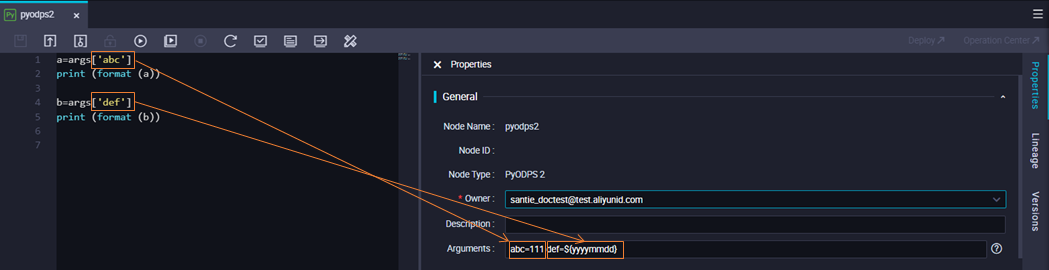• In the following example, a common Shell node is used.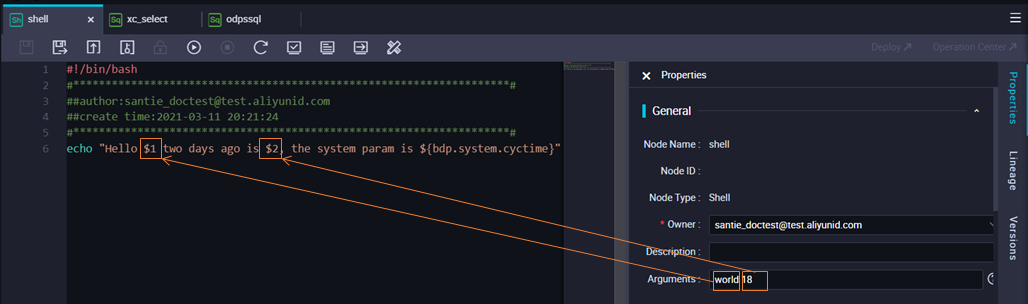You are not allowed to customize variable names for common Shell nodes. The variable names must follow the \$1,\$2,\$3, ...format. If the number of parameters reaches 10, use \${10} to declare the tenth variable.

## Test scheduling parameters in the development environment

If you use scheduling parameters during data development, you can perform smoke testing on the DataStudio page to check whether the values of the parameters meet your expectations. During the smoke testing, you must manually replace the parameters with constants.
• Perform smoke testing
You can click Perform Smoke Testing in Development Environment in the toolbar of the node configuration tab. Then, enter the data timestamp to simulate the scenario in which the node is scheduled to run. Obtain the values of the scheduling parameters after the parameters are replaced, and check whether the values meet your expectations based on the smoke testing result.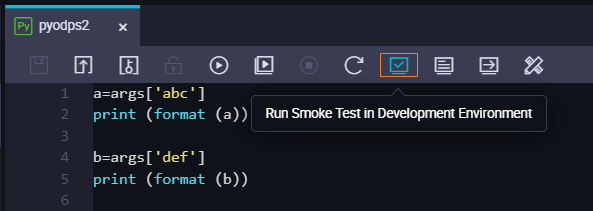Note
• If you modify the scheduling parameters when you perform smoke testing for a node in the development environment, save and commit the node in the development environment again. Otherwise, the code cannot be updated.
• When you perform smoke testing in the development environment, you are charged for the test instances that are generated.
• Manually replace scheduling parameters
After you click Run (1) or Run with Parameters (2) on the node configuration tab, scheduling parameters are not automatically replaced with the variables specified on the Properties tab. You must manually assign constants to the variables (3 and 4) in the code.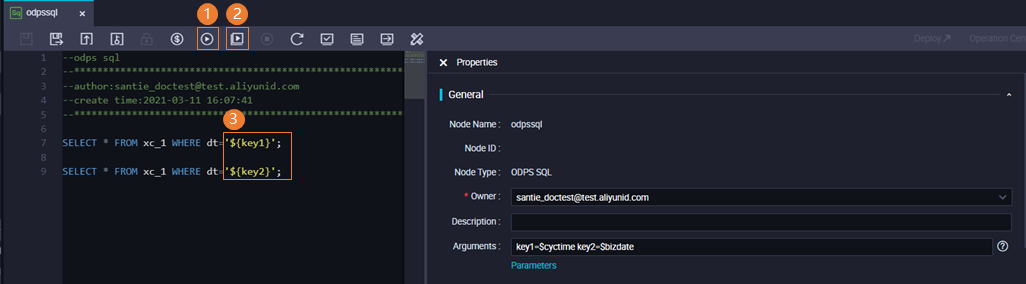## View the parameter replacement results in Operation Center

After an auto triggered node is developed, committed, and deployed, the scheduling parameters of node instances are replaced when the instances are generated. You can check whether the scheduling parameters are replaced as expected on the Cycle Task page. To go to this page, choose Cycle Task Maintenance > Cycle Task in the left-side navigation pane of the Operation Center page.

For example, you modify the scheduling parameters of an auto triggered node on the DataStudio page, commit the node, and then deploy it in the production environment. In this case, you must check whether the parameter values in the Execution Parameters field meet your expectations.